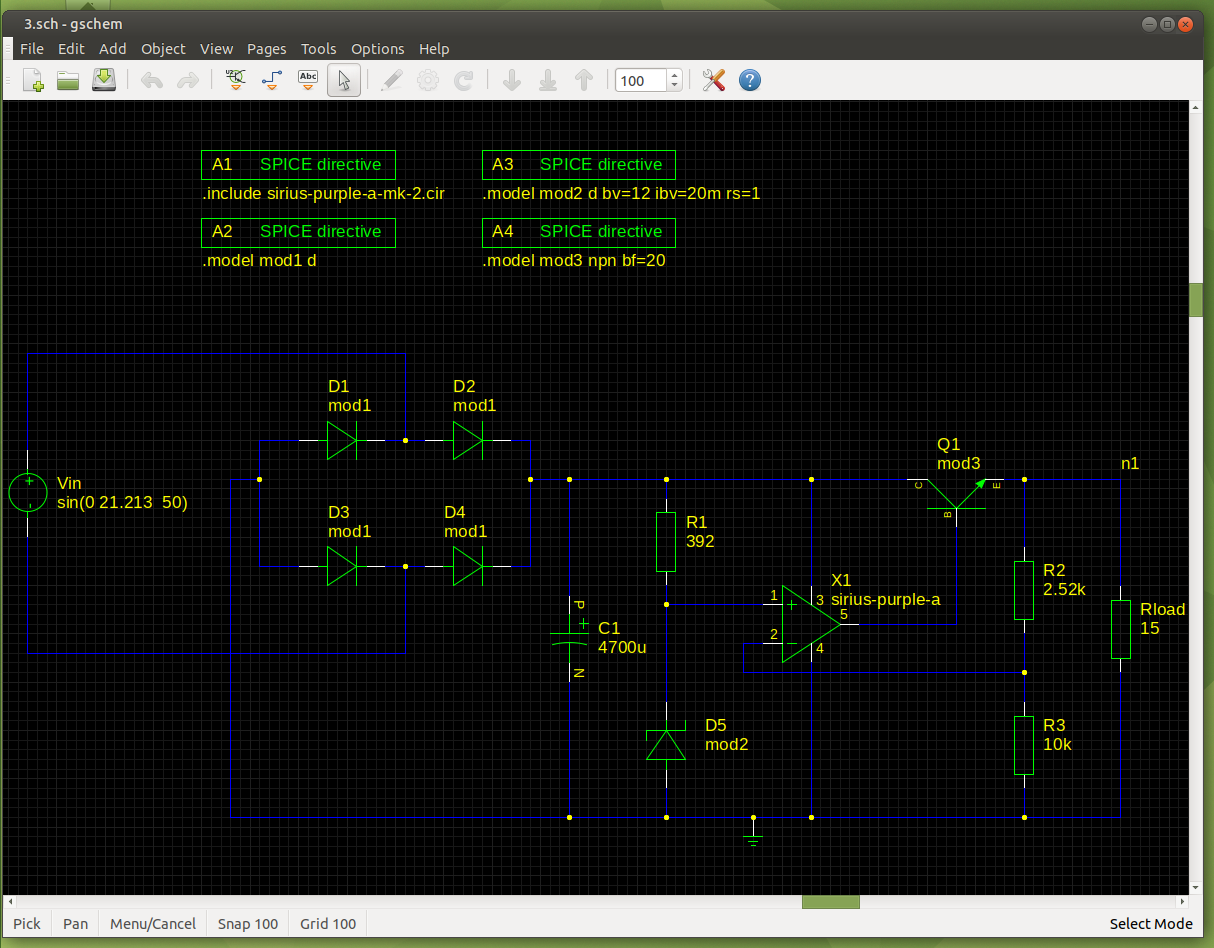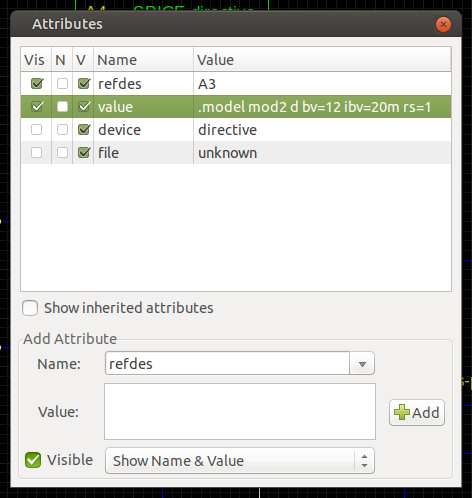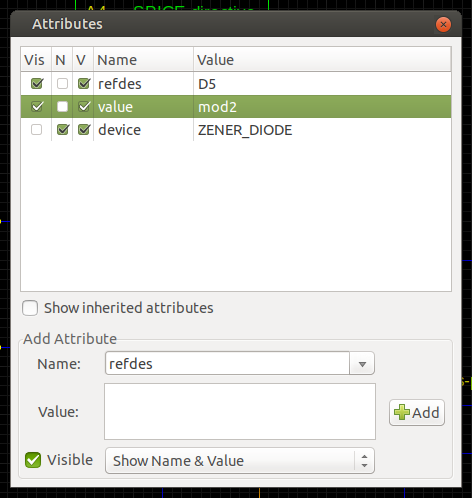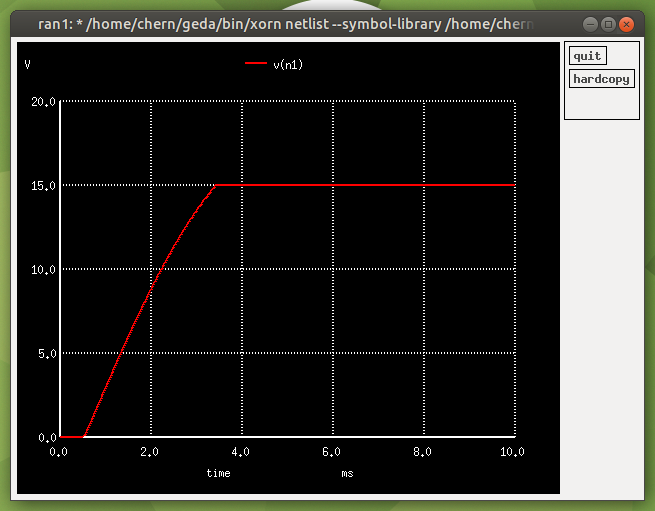# Zener DiodesTo make a Zener diode, select zener-1.sym in gschem, found under "Diodes (generic)". Change its refdes from Z to D. For the model, I used the default SPICE diode model (d). Then enter its reverse breakdown voltage (I used bv=12) at the end of the define model SPICE directive (A3 in my schematic). You can also enter its current at reverse breakdown voltage and series resistance (point 10 on the web page). The current at reverse breakdown voltage ibv is Iz (or Itest) on the datasheet. A typical value for the series resistance rs is 1Ω:Then change the "value" of the Zener diode to the name of the model (I used mod2):Above is an example of an Operational Amplifier Linear Regulator.

The input voltage Vin is 15Vrms (21.213Vpeak), 50Hz. The load current is assumed to be 1A, so the load resistor is Vout/1A = 15V/1A = 15Ω.

Now for a simulation!

Transient analysis:We can see that the voltage is regulated to 15V!